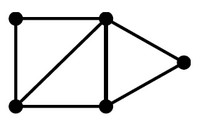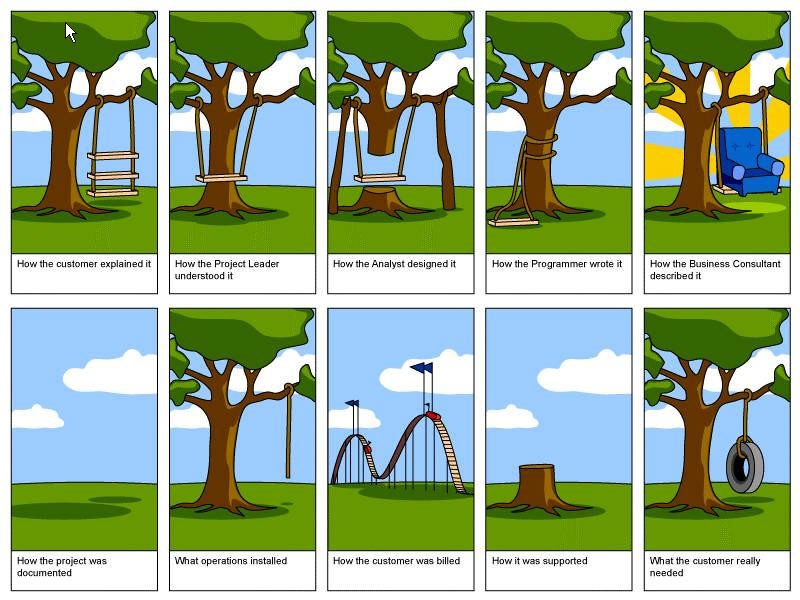# Graph - Graph Model (Network Model)

Logical Data Modeling - Data Model for a graph.

A graph data model is also known as a wiki/Network model

The schema is composed:

## Element

The property graph contains:

## Documentation / Reference

Recommended PagesGraph (Network - Nodes and edges)

A graph is a set of vertices connected by edges. See Data representation that naturally captures complex relationships is a graph (or network). Except of the special graph that a tree is, the data...Graph - (Edge | Links | Arcs | Lines | Arrows) - Association

An edge model a relationship betweentwo node in a graph. Every edge model therefore a binary relationship (relationship between two elements) . An Edge is also known as: Links Arcs Lines Arrows...Graph - (Node | Vertice | Vertex | Point)

A node in a graph model an entity The model graph contains connected the nodes: containing any number of attributes (key-value-pairs). tagged with labels (to label, attach metadata—​index or...Logical Data Modeling - Data Model

A data model is an (abstract) model that describes how data are represented and accessed. Data models formally define entity and relationships among entities for a domain of interest. schema A data...